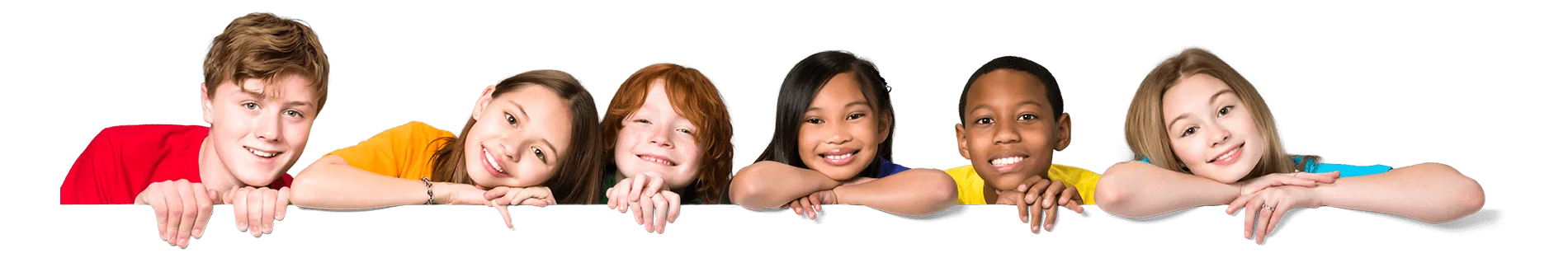Latest Results:

# 11plus Algebra sample papers

Solve this Question

#### If X + Y = 200 and X – Y = 100, then value of  Y is ______________

Solve this Question

#### Solve the equation. 2x - 2 + 3x = -4 + 2x + 14

 1. -4 2. -3 3. 4 4. 3

Solve this Question

#### Represent the following in the form of equation.9 more than the cube of a number ‘y’ is equal to the product of four and number ‘x’.

 1. \({\ {9+y^3=4x} }\) 2. \({\ {9+4x=y^3} }\) 3. \({\ {9+y^3=4+x} }\) 4. \({\ {y^3=4x+x+9} }\)

Solve this Question

#### By understanding the following conversation between Robert and John, answer the question.Robert: “How many hens do you have in your farm?”John:”Twice the number of hens is same as one more than the square of number of hens”.Find the number of hens.

 1. 1 2. 2 3. 3 4. 4